# Examples - Photochemistry Chemistry Notes | EduRev

## Chemistry : Examples - Photochemistry Chemistry Notes | EduRev

The document Examples - Photochemistry Chemistry Notes | EduRev is a part of the Chemistry Course Physical Chemistry.
All you need of Chemistry at this link: Chemistry

Problem. The carbon-14 activity of an old wood sample is found to be 14.2 disintegration min–1 g–1. Calculate age of old wood sample, if for a fresh wood sample carbon-14 activity is 15.3 disintegration min–1 g–1 (t1/2 carbon =14) = 5730 year), is

(1) 5000 year (2) 4000 year (3) 877 year (4) 617 year

Sol.     Act ivit y =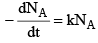k = rate constant
and    NA = no. of atom
Activity of old wood = k Nold = 14.2 …(1)
Activity of new wood = k Nnew = 15.3 …(2)

From equation (1) & (2) we get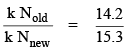or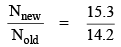…(3)

We know that k × t1/2 = 0.693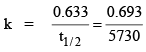…(4)

We know that

i.e.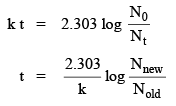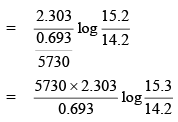T = 617 year

Problem. Using cuvettes of 0.5 cm path length, a 10–4 M solution of a chromphone shows 50% transmittance at certain wave length. The molar extinction coefficient of the chromphre at this wave length is (log 2 = 0.3010)

(1) 1500 M–1 cm–1 (2) 3010 M–1 cm–1 (3) 5000 M–1 cm–1 (4) 6020 M–1 cm–1

Sol.

Transmittance = T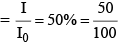Absorbance = A =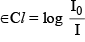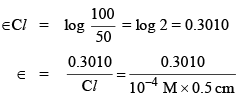∈= 6020 M–1 cm–1

Problem. The rate law for one of the mechanisms of the pyrolysis of CH3CHO at 520°C and 0.2 bar is:
Rate =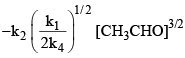The overall activation energy Ein terms of the rate law is:

(1) Ea(2) + Ea(1) + 2Ea(4)   (2)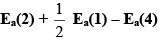(3)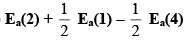(4)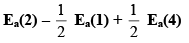Sol.    Rate =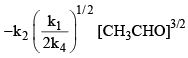= koverall [CH3CHO]3/2
i.e.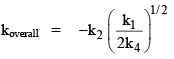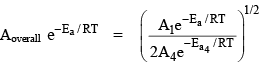i.e.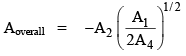and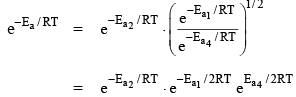or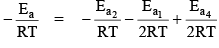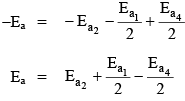Problem. In the Michaelis-Menten mechanism of enzyme kinetics, the expression obtained as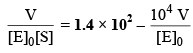The value of k3 and k(Michaelis constant, mol L–1) are

(1) 1.4 × 1012, 104 (2)1.4 × 108, 104 (3) 1.4 × 108, 10–4 (4) 1.4 × 1012, 10–4

Sol.  We know that Michaelis Menten equation is: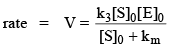…(1)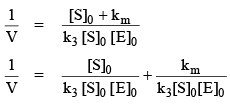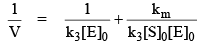Multiply this equation by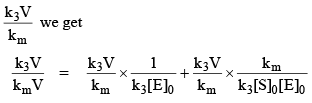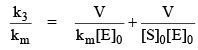or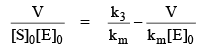…(2)

and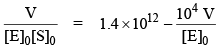…(3)

Comparing equation (2) & (3) we get

i.e.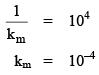and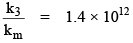k3 = 1.4 × 1012 × 10–4

= 1.4 × 108
i.e. The correct answer is (3).

Problem. The Langunier adsorption isotherm is given by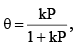where P is the pressure of the adsorbate gas. The Langmuir adsorption isotherm for a diatomic gas Aundergoing dissociative  adsorption is

(1)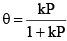(2)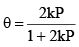(3)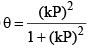(4)Sol.  R(g) + M(surface)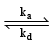RM (surface)

then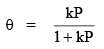if  R2(g) + 2M (surface)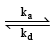2M (surface)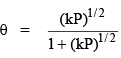i.e. the correct answer is (4).

Problem. The overall rate of following complex reaction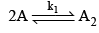(fast equilibrium)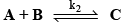(fast equilibrium)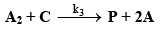(slow)

The steady state approximate would be

(1) k1k2k3[A]3[B]

(2) k1k2k3[A][B]3

(3) k1k2k3[A][B]2

(4) k1k2k3[A][B]

Sol.(fast equilibrium)

then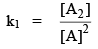…(1)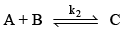(fast equilibrium)

then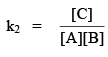…(2)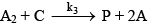(slow)

The rate of formation of product P is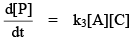…(3)

From equation (1) & (2) we get

&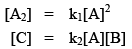then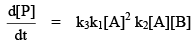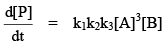i.e. the correct answer is (1)

Problem. The species 19Ne and 14C emit a position and b-particle respectively. The resulting species formed are respectively

(1) 19Na and 14B (2) 19F and 14N (3) 19Na and 14N (4) 19F and 14

Sol.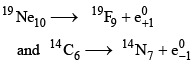i.e. the correct answer is (b).

Problem. The half life of a zero order reaction (A → P) is given by (k = rate constant)

(1)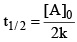(2)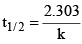(3)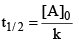(4)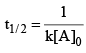Sol.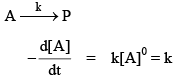if t = t1/2 then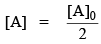Thus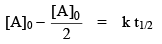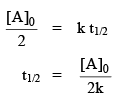i.e. the correct answer is (1).

Offer running on EduRev: Apply code STAYHOME200 to get INR 200 off on our premium plan EduRev Infinity!

## Physical Chemistry

77 videos|83 docs|32 tests

,

,

,

,

,

,

,

,

,

,

,

,

,

,

,

,

,

,

,

,

,

;